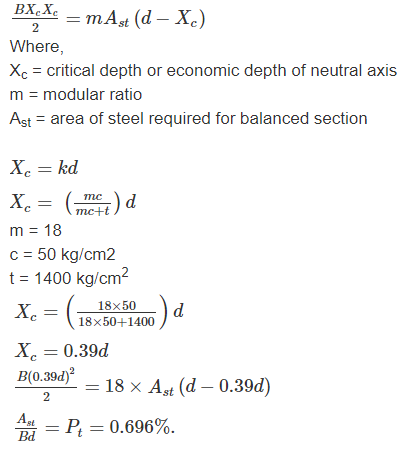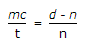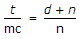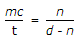# Civil Engineering - RCC Structures Design

6.
If the permissible compressive and tensile stresses in a singly reinforced beam are 50 kg/cm2 and 1400 kg/cm2 respectively and the modular ratio is 18, the percentage area At of the steel required for an economic section, is
0.496%
0.596%
0.696%
0.796%
none of these.
Explanation:

Taking Moment of area of tension and compression zone for a singly reinforced beam:7.
The live load to be considered for an inaccessible roof, is
Nil
75 kg/m2
150 kg/cm2
200 kg/m2
Explanation:
No answer description is available. Let's discuss.

8.
If the maximum shear stress at the end of a simply supported R.C.C. beam of 6 m effective span is 10 kg/cm2, the share stirrups are provided for a distance x from either end where x is
50 cm
100 cm
150 cm
200 cm
Explanation:
No answer description is available. Let's discuss.

9.
The radius of a bar bend to form a hook, should not be less than
twice the diameter
thrice the diameter
four times the diameter
five times the diameter
none of these.